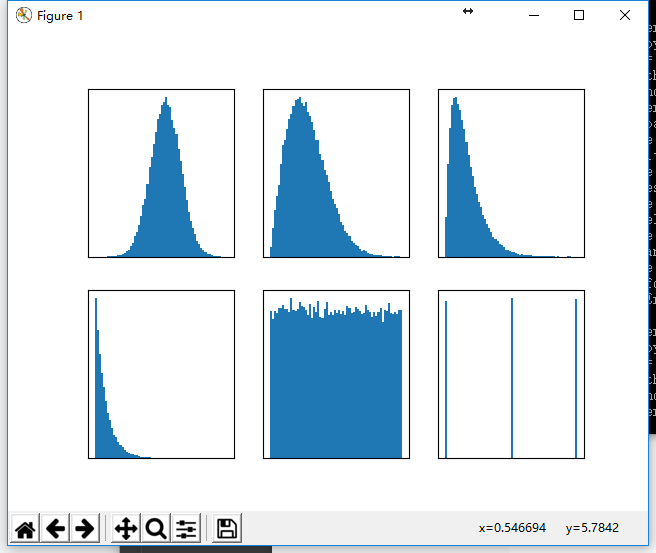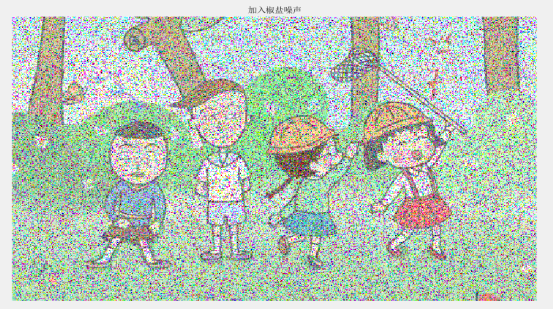2017-12-15 11:01:55 vitaminc4 阅读数 1161
• ###### 机器学习算法实战——神秘奥妙的支持向量机

支持向量机算法是机器学习的重要算法，如今已应用在图像处理、 语音识别和自然语言处理等方面。本课程详细讲解支持向量机的原理、相关概念、 推导过程和代码实战。包括：Logistic函数、最大化间隔、凸二次优化、核函数、 数据中的噪声点处理等知识。最后用了手写字分类实例，详细讲解了相关的代码实战。

47 人正在学习 去看看 穆辉宇

### 椒盐噪声：

2019-02-22 17:06:56 qq_34725005 阅读数 103
• ###### 机器学习算法实战——神秘奥妙的支持向量机

支持向量机算法是机器学习的重要算法，如今已应用在图像处理、 语音识别和自然语言处理等方面。本课程详细讲解支持向量机的原理、相关概念、 推导过程和代码实战。包括：Logistic函数、最大化间隔、凸二次优化、核函数、 数据中的噪声点处理等知识。最后用了手写字分类实例，详细讲解了相关的代码实战。

47 人正在学习 去看看 穆辉宇
``````import matplotlib.pyplot as plt
import numpy as np

#高斯噪声：均值为0，标准差为64
x1 = np.random.normal(loc=0, scale=64, size=(256, 256))
#瑞利噪声：（2/b）**0.5为1
x2 = np.random.rayleigh(scale=64, size=(256, 256))
#伽马噪声：（b-1）/a为2，放大32倍
x3 = np.random.gamma(shape=2, scale=32, size=(256, 256))
#指数噪声：a=1/32
x4 = np.random.exponential(scale=32, size=(256, 256))
#均匀噪声
x5 = np.random.uniform(low=0, high=1.0, size=(256, 256))
#脉冲噪声
x6 = np.random.random_integers(low=0, high=2.0, size=(256, 256))

for i, x in enumerate([x1, x2, x3, x4, x5, x6]):
ax = plt.subplot(23 * 10 + i + 1)
ax.hist(x.reshape(x.size), 64, normed=True)
ax.set_yticks([])
ax.set_xticks([])
plt.show()
``````2019-12-20 10:36:34 rong11417 阅读数 161
• ###### 机器学习算法实战——神秘奥妙的支持向量机

支持向量机算法是机器学习的重要算法，如今已应用在图像处理、 语音识别和自然语言处理等方面。本课程详细讲解支持向量机的原理、相关概念、 推导过程和代码实战。包括：Logistic函数、最大化间隔、凸二次优化、核函数、 数据中的噪声点处理等知识。最后用了手写字分类实例，详细讲解了相关的代码实战。

47 人正在学习 去看看 穆辉宇

1.介绍

2.模拟图像处理

3.数字图像处理

4.什么是图像

5.数字图像和信号之间的关系

6.如何形成数字图像

7.应用

## 4.什么是图像128 30 123 232 123 321 123 77 89 80 255 255

## 7.应用

### 机器/计算机视觉### 计算机图形学### 信号处理

2019-07-02 20:57:49 qq_37486501 阅读数 1054
• ###### 机器学习算法实战——神秘奥妙的支持向量机

支持向量机算法是机器学习的重要算法，如今已应用在图像处理、 语音识别和自然语言处理等方面。本课程详细讲解支持向量机的原理、相关概念、 推导过程和代码实战。包括：Logistic函数、最大化间隔、凸二次优化、核函数、 数据中的噪声点处理等知识。最后用了手写字分类实例，详细讲解了相关的代码实战。

47 人正在学习 去看看 穆辉宇

### 噪声分类:

• 高斯噪声
1. 是随机噪声, 服从高斯分布
2. 主要特点表现为:麻点
• 椒盐噪声
1. 胡椒噪声、盐噪声
2. 主要特点表现为:黑白点

### 噪声的描述

1. 均方误差 MSE : MSE越大,失真率越大
2. 峰值信噪比 PSNR: PSNR越大,失真度越小

### 图像平滑(去噪)

1. 平滑的目的: 在表刘源是图像基本特征的前提下, 消除或衰减噪声的影响, 提高视觉效果

2. 基础知识:
(1): 滤波: 使用空间模版(滤波器)处理图像的过程
(2): 模版与模版运算: 模版和邻域大小相同

3. 常用图像平滑方法(空间平滑滤波):

• 均值滤波 (邻域平均法)——线性空间滤波
`MATLAB实现均值滤波,见我的博客:`
https://blog.csdn.net/qq_37486501/article/details/80274928
(1): 基本思想: 某像素点灰度值=邻域中所有像素灰度值平均值 来代替
(2): 优点: 在一定程度上可衰减噪声影响——拉小灰度差异
(3): 缺点: 图像的边缘轮廓细节变模糊——边缘轮廓也做均值,导致的变模糊

• 中值滤波(中位数)——非线性滤波
`MATLAB实现中值滤波,见我的博客:`
https://blog.csdn.net/qq_37486501/article/details/80274960
(1): 基本思想: 某像素的灰度值=窗口内所有像素的灰度中值 来代替
(2):窗口:
有不同形状(
一维:线状
二维:十字、正方形、菱形、圆形)
有不同大小(窗口大小中必含奇数元素, 为了保证中心像素值)
(3): 优点:
在去噪同时,较好的保持边缘轮廓细节
适合处理椒盐噪声(因为: 不是去噪声点, 而使改变其灰度值)

• 小波去噪
将信号通过小波变换（采用Mallat算法）后，信号产生的小波系数含有信号的重要信息，将信号经小波分解后小波系数较大，噪声的小波系数较小，并且噪声的小波系数要小于信号的小波系数，通过选取一个合适的阀值，大于阀值的小波系数被认为是有信号产生的，应予以保留，小于阀值的则认为是噪声产生的，置为零从而达到去噪的目的。

• 高斯滤波
高斯滤波是一种线性平滑滤波，适用于消除高斯噪声，广泛应用于图像处理的减噪过程。高斯滤波就是对整幅图像进行加权平均的过程，每一个像素点的值，都由其本身和邻域内的其他像素值经过加权平均后得到。高斯滤波的具体操作是：用一个模板（或称卷积、掩模）扫描图像中的每一个像素，用模板确定的邻域内像素的加权平均灰度值去替代模板中心像素点的值。

• 双边滤波器去噪
双边滤波器（Bilateral filter）是一种可以保边去噪的滤波器。可以滤除图像数据中的噪声，且还会保留住图像的边缘、纹理等（因噪声是高频信号，边缘、纹理也是高频信息，高斯滤波会在滤除噪声的同时使得边缘模糊）。是使用一个卷积核（模板矩阵），叠加到待处理像素点上，使用对应邻域像素点的加权求和来作为新的输出像素点的值一种方法，简单来说，双边滤波和高斯滤波一样，不同只在于模板矩阵的不同。

2019-12-12 22:28:47 weixin_44225182 阅读数 156
• ###### 机器学习算法实战——神秘奥妙的支持向量机

支持向量机算法是机器学习的重要算法，如今已应用在图像处理、 语音识别和自然语言处理等方面。本课程详细讲解支持向量机的原理、相关概念、 推导过程和代码实战。包括：Logistic函数、最大化间隔、凸二次优化、核函数、 数据中的噪声点处理等知识。最后用了手写字分类实例，详细讲解了相关的代码实战。

47 人正在学习 去看看 穆辉宇

1.熟悉MATLAB软件的使用。
2.掌握图像的噪声与滤波处理。

## 三、实验内容

1.对一张图片添加不同强度的高斯噪声、椒盐噪声
2.对图像进行不同模板的均值滤波、高斯加权滤波、中值滤波，对比结果并分析不同滤波方式的优劣

Win10 64位电脑
MATLAB2017a

## 五、实验原理

所谓高斯噪声是指它的概率密度函数服从高斯分布（即正态分布）的一类噪声。如果一个噪声，它的幅度分布服从高斯分布，而它的功率谱密度又是均匀分布的，则称它为高斯白噪声。高斯白噪声的二阶矩不相关，一阶矩为常数，是指先后信号在时间上的相关性。高斯白噪声包括热噪声和散粒噪声。在通信信道测试和建模中，高斯噪声被用作加性白噪声以产生加性白高斯噪声。

椒盐噪声也称为脉冲噪声，是图像中经常见到的一种噪声，它是一种随机出现的白点或者黑点，可能是亮的区域有黑色像素或是在暗的区域有白色像素（或是两者皆有）。盐和胡椒噪声的成因可能是影像讯号受到突如其来的强烈干扰而产生、类比数位转换器或位元传输错误等。例如失效的感应器导致像素值为最小值，饱和的感应器导致像素值为最大值。

均值滤波也称为线性滤波，其采用的主要方法为邻域平均法。线性滤波的基本原理是用均值代替原图像中的各个像素值，即对待处理的当前像素点（x，y），选择一个模板，该模板由其近邻的若干像素组成，求模板中所有像素的均值，再把该均值赋予当前像素点（x，y），作为处理后图像在该点上的灰度g（x，y），即g（x，y）=∑f（x，y）/m m为该模板中包含当前像素在内的像素总个数。

高斯滤波实质上是一种信号的滤波器，其用途是信号的平滑处理，人们知道数字图像用于后期应用，其噪声是最大的问题，由于误差会累计传递等原因，很多图像处理教材会在很早的时候介绍Gauss滤波器，用于得到信噪比SNR较高的图像（反应真实信号）。与此相关的有Gauss-Laplace变换，其实就是为了得到较好的图像边缘，先对图像做Gauss平滑滤波，剔除噪声，然后求二阶导矢，用二阶导的过零点确定边缘，在计算时也是频域乘积至空域卷积。

中值滤波是基于排序统计理论的一种能有效抑制噪声的非线性信号处理技术，中值滤波的基本原理是把数字图像或数字序列中一点的值用该点的一个邻域中各点值的中值代替，让周围的像素值接近的真实值，从而消除孤立的噪声点。方法是用某种结构的二维滑动模板，将板内像素按照像素值的大小进行排序，生成单调上升（或下降）的为二维数据序列。二维中值滤波输出为g（x,y）=med{f(x-k,y-l),(k,l∈W)} ，其中，f(x,y)，g(x,y)分别为原始图像和处理后图像。W为二维模板，通常为33，55区域，也可以是不同的的形状，如线状，圆形，十字形，圆环形等。

## 六、实验过程及代码

``````t=imread('a1.jpg');
imshow(t),title('原图');
t1=imnoise(t,'gaussian',0,0.01);
figure,imshow(t1),title('添加均值为0，方差为0.01的高斯噪声');
t2=imnoise(t,'gaussian',0,0.02);
figure,imshow(t2),title('添加均值为0，方差为0.02的高斯噪声');
t3=imnoise(t,'gaussian',0,0.03);
figure,imshow(t3),title('添加均值为0，方差为0.03的高斯噪声');
t4=imnoise(t,'gaussian',0.2,0.01);
figure,imshow(t4),title('添加均值为0.2，方差为0.01的高斯噪声');
t5=imnoise(t,'gaussian',0.4,0.01);
figure,imshow(t5),title('添加均值为0.4，方差为0.01的高斯噪声');
``````

``````t=imread('a1.jpg');
[m,n,z]=size(t);
y=0+0.1*randn(m,n);
t1=double(t)/255;
t1=t1+y;
t1=t1*255;
t1=uint8(t1);
subplot(1,2,1),imshow(t),title('原图');
subplot(1,2,2),imshow(t1),title('加入均值为0，标准差为0.1的高斯噪声后');
``````

`````` image=imread('a1.jpg');
[width,height,z]=size(image);
subplot(1,2,1);
imshow(image);
title('原图');
av=0;
std=0.1;
u1=rand(width,height);
u2=rand(width,height);
x=std*sqrt(-2*log(u1)).*cos(2*pi*u2)+av;
result1=double(image)/255+x;
result1=uint8(255*result1);
subplot(1,2,2);
imshow(result1);
title('加入均值为0，标准差为0.1的高斯噪声后');
``````

`````` t=imread('a1.jpg');
subplot(1,2,1),imshow(t),title('原图');
t1=imnoise(t,'salt & pepper',0.1);
subplot(1,2,2),imshow(t1),title('加入噪声密度：0.1的椒盐噪声');
t2=imnoise(t,'salt & pepper',0.2);
figure,subplot(1,2,1),imshow(t2),title('加入噪声密度：0.2的椒盐噪声');
t3=imnoise(t,'salt & pepper',0.3);
subplot(1,2,2),imshow(t3),title('加入噪声密度：0.3的椒盐噪声');
``````

``````image=imread('a1.jpg');
[width,height,z]=size(image);

result2=image;
subplot(1,2,1)
imshow(image);
title('原图');
k1=0.2;
k2=0.2;
a1=rand(width,height)<k1;
a2=rand(width,height)<k2;
t1=result2(:,:,1);
t2=result2(:,:,2);
t3=result2(:,:,3);
t1(a1&a2)=0;
t2(a1&a2)=0;
t3(a1&a2)=0;
t1(a1& ~a2)=255;
t2(a1& ~a2)=255;
t3(a1& ~a2)=255;
result2(:,:,1)=t1;
result2(:,:,2)=t2;
result2(:,:,3)=t3;
subplot(1,2,2)
imshow(result2);
title('加高斯噪声后');
``````

`````` t=imread('a1.jpg');
[m,n,z]=size(t);
t1=imnoise(t,'gaussian',0,0.02);
imshow(t1),title('加入高斯噪声后')
t2=t;
for i=1:m-2
for j=1:n-2
t2(i+1,j+1,:)=round(t2(i,j,:)/9+t2(i,j+1,:)/9+t2(i,j+2,:)/9+t2(i+1,j,:)/9+t2(i+1,j+1,:)/9+t2(i+1,j+2,:)/9+t2(i+2,j,:)/9+t2(i+2,j+1,:)/9+t2(i+2,j+2,:)/9);
end
end
figure,
subplot(1,2,1),imshow(t2),title('3*3均值滤波');
t3=t;
for i=1:m-4
for j=1:n-4
t3(i+2,j+2,:)=round(t3(i,j,:)/25+t3(i,j+1,:)/25+t3(i,j+2,:)/25+t3(i,j+3,:)/25+t3(i,j+4,:)/25+t3(i+1,j,:)/25+t3(i+1,j+1,:)/25+t3(i+1,j+2,:)/25+t3(i+1,j+3,:)/25+t3(i+1,j+4,:)/25+t3(i+2,j,:)/25+t3(i+2,j+1,:)/25+...
t3(i+2,j+2,:)/25+t3(i+2,j+3,:)/25+t3(i+2,j+4,:)/25+t3(i+3,j,:)/25+t3(i+3,j+1,:)/25+t3(i+3,j+2,:)/25+t3(i+3,j+3,:)/25+t3(i+3,j+4,:)/25+...
t3(i+4,j,:)/25+t3(i+4,j+1,:)/25+t3(i+4,j+2,:)/25+t3(i+4,j+3,:)/25+t3(i+4,j+4,:)/25);
end
end
subplot(1,2,2),imshow(t3),title('5*5均值滤波');
t1=imnoise(t,'salt & pepper',0.3);
figure,imshow(t1),title('加入椒盐噪声后')
t2=t;
for i=1:m-2
for j=1:n-2
t2(i+1,j+1,:)=round(t2(i,j,:)/9+t2(i,j+1,:)/9+t2(i,j+2,:)/9+t2(i+1,j,:)/9+t2(i+1,j+1,:)/9+t2(i+1,j+2,:)/9+t2(i+2,j,:)/9+t2(i+2,j+1,:)/9+t2(i+2,j+2,:)/9);
end
end
figure,
subplot(1,2,1),imshow(t2),title('3*3均值滤波');
t3=t;
for i=1:m-4
for j=1:n-4
t3(i+2,j+2,:)=round(t3(i,j,:)/25+t3(i,j+1,:)/25+t3(i,j+2,:)/25+t3(i,j+3,:)/25+t3(i,j+4,:)/25+t3(i+1,j,:)/25+t3(i+1,j+1,:)/25+t3(i+1,j+2,:)/25+t3(i+1,j+3,:)/25+t3(i+1,j+4,:)/25+t3(i+2,j,:)/25+t3(i+2,j+1,:)/25+...
t3(i+2,j+2,:)/25+t3(i+2,j+3,:)/25+t3(i+2,j+4,:)/25+t3(i+3,j,:)/25+t3(i+3,j+1,:)/25+t3(i+3,j+2,:)/25+t3(i+3,j+3,:)/25+t3(i+3,j+4,:)/25+...
t3(i+4,j,:)/25+t3(i+4,j+1,:)/25+t3(i+4,j+2,:)/25+t3(i+4,j+3,:)/25+t3(i+4,j+4,:)/25);
end
end
subplot(1,2,2),imshow(t3),title('5*5均值滤波');
``````

``````function h = gaussian( hsize, sigma)
siz = (hsize-1)/2;
[x,y] = meshgrid(-siz(2):siz(2),-siz(1):siz(1));
arg = -(x.*x + y.*y)/(2*sigma*sigma);
h = exp(arg);
h(h<eps*max(h(:))) = 0;
sumh = sum(h(:));
if sumh ~= 0
h = h/sumh;
end
end

[m,n,z]=size(t);
t1=imnoise(t,'gaussian',0,0.02);
imshow(t1),title('加入高斯噪声后');
martex=gaussian([3 3],1);
t2=t;
for i=m-2
for j=n-2
t2(i+1,j+1,:)=t2(i,j,:)*martex(1,1)+t2(i,j+1,:)*martex(1,2)+t2(i,j+2,:)*martex(1,3)+t2(i+1,j,:)*martex(2,1)+...
t2(i+1,j+1,:)*martex(2,2)+t2(i+1,j+2,:)*martex(2,3)+t2(i+2,j,:)*martex(3,1)+t2(i+2,j+1,:)*martex(3,2)+t2(i+2,j+2,:)*martex(3,3);
end
end
figure,imshow(t2),title('高斯滤波')
``````

``````function [ img ] = median_filter( image, m )
n = m;
[ height, width ] = size(image);
x1 = double(image);
x2 = x1;
for i = 1: height-n+1
for j = 1:width-n+1
mb = x1( i:(i+n-1),  j:(j+n-1) );
mb = mb(:);
mm = median(mb);
x2( i+(n-1)/2,  j+(n-1)/2 ) = mm;

end
end

img = uint8(x2);
end

t1=imnoise(t,'salt & pepper',0.3);
imshow(t1),title('加入椒盐噪声');
t2=median_filter(t,3);
figure,imshow(t),title('中值滤波后')
``````

## 七、实验结果与分析1.均值决定图像的整体灰度范围，随着均值的增大，那么图像整体的灰度同样也会增长，当然，超过255依然为255，但更多的像素靠近255，从视觉上看起来更加偏白，偏亮。
2.方差决定着图像噪声的密集程度及概率分布程度，方差越大，图像中的噪声对图片的影响也就越大，从视觉上看就是更加密集。